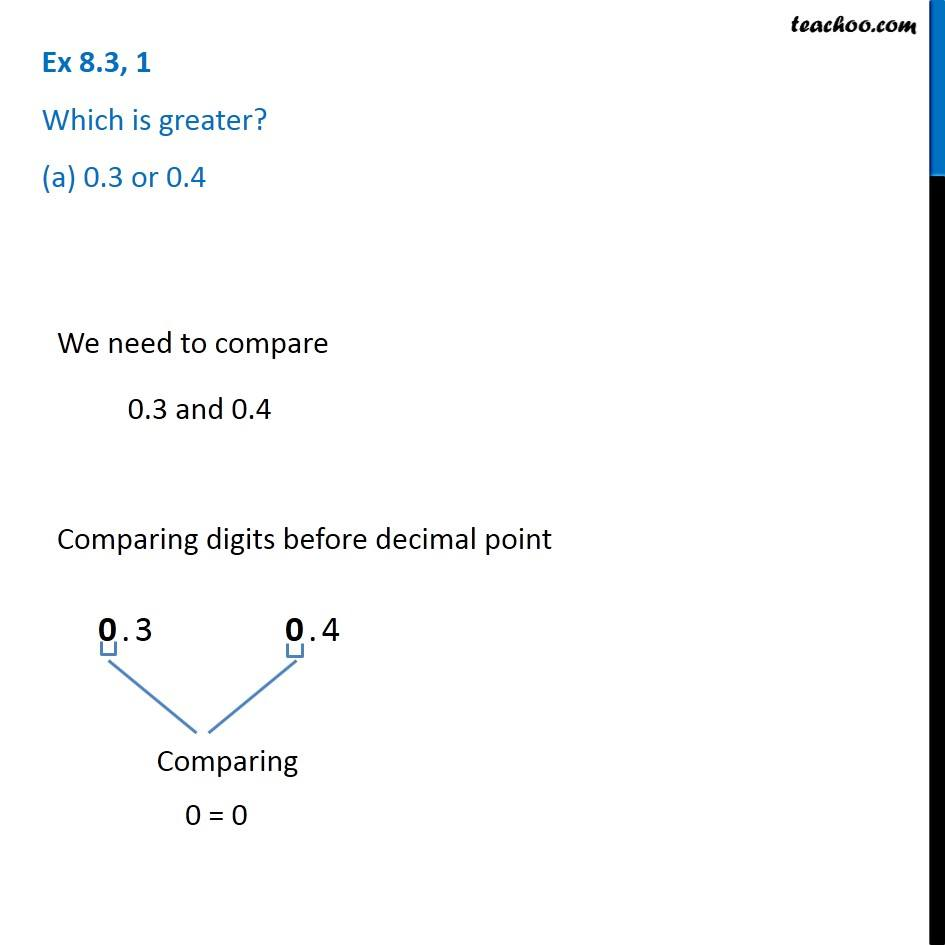1. Chapter 8 Class 6 Decimals
2. Concept wise
3. Comparing decimals

Transcript

Ex 8.3, 1 Which is greater? (a) 0.3 or 0.4 We need to compare 0.3 and 0.4 Comparing digits before decimal point 0 = 0 So, we compare digits after decimal point 3 < 4 So, 0.3 < 0.4 Thus, 0.4 is greater

Comparing decimals